# Regular boundary point

(diff) ← Older revision | Latest revision (diff) | Newer revision → (diff)

A point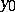on the boundaryof a domainin a Euclidean space,, at which, for any continuous functionon, the generalized solution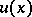of the Dirichlet problem in the sense of Wiener–Perron (see Perron method) takes the boundary value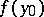, that is,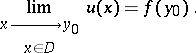The regular boundary points ofform a set, at the points of which the complementis not a thin set; the set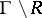of irregular boundary points (cf. Irregular boundary point) is a polar set of type. If all points ofare regular boundary points, then the domainis called regular with respect to the Dirichlet problem.

Forto be a regular boundary point it is necessary and sufficient that in the intersectionofwith any neighbourhoodofthere is a superharmonic barrier (a functionin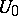such that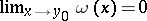, Lebesgue's criterion for a barrier). It was first shown by H. Lebesgue in 1912 that forthe vertex of a sufficiently acute angle lying inneed not be a regular boundary point.

Let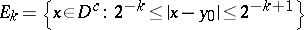and let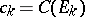be the capacity of the set. Forto be a regular boundary point it is necessary and sufficient that the series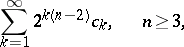diverges, or for, that the series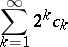diverges, where(Wiener's criterion).

For, a point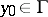is a regular boundary point if there is a continuous path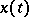,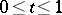, such that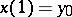, and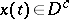for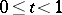. When, a pointis a regular boundary point if it can be reached by the vertex of a right circular cone belonging to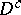in a sufficiently small neighbourhood of. In the case of a domainin the compactified space,, the point at infinity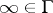is always a regular boundary point; when, the point at infinityis a regular boundary point if there is a continuous path,, such thatfor, and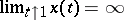.

How to Cite This Entry:
Regular boundary point. Encyclopedia of Mathematics. URL: http://encyclopediaofmath.org/index.php?title=Regular_boundary_point&oldid=15319
This article was adapted from an original article by E.D. Solomentsev (originator), which appeared in Encyclopedia of Mathematics - ISBN 1402006098. See original article